Gao, Y., Hua, J., Chen, G., Cai, L., Jia, N., and Zhu, L. (2019). "Bi-directional prediction of wood fiber production using the combination of improved particle swarm optimization and support vector machine," BioRes. 14(3), 7229-7246.

#### Abstract

In order to investigate the relationship between production parameters and evaluation indexes for wood fiber production, a bi-directional prediction model was established to predict the fiber quality, energy consumption, and production parameters based on the improved particle swarm optimization and support vector machine (IPSO-SVM). SVM was applied to build the bi-directional prediction model, and IPSO was used to optimize the SVM parameters that affect the performance of prediction greatly. In the case of the forward prediction, the model can predict the fiber quality and energy consumption using the production parameters as input information; in the case of the backward prediction, the model can estimate production parameters using the fiber quality and energy consumption as evaluation indexes for input information. The results showed that the IPSO-SVM model had high accuracy and good generalization ability in the prediction for the wood fiber production. Additionally, based on the effectiveness of the proposed model and preset evaluation indexes, the corresponding production parameters were determined, which was able to save the wooden resources as well as reduce energy consumption in the fiberboard production.

Bi-directional Prediction of Wood Fiber Production Using the Combination of Improved Particle Swarm Optimization and Support Vector Machine

Yunbo Gao,a Jun Hua,a,* Guangwei Chen,a Liping Cai,b,c Na Jia,a and Liangkuan Zhu a

In order to investigate the relationship between production parameters and evaluation indexes for wood fiber production, a bi-directional prediction model was established to predict the fiber quality, energy consumption, and production parameters based on the improved particle swarm optimization and support vector machine (IPSO-SVM). SVM was applied to build the bi-directional prediction model, and IPSO was used to optimize the SVM parameters that affect the performance of prediction greatly. In the case of the forward prediction, the model can predict the fiber quality and energy consumption using the production parameters as input information; in the case of the backward prediction, the model can estimate production parameters using the fiber quality and energy consumption as evaluation indexes for input information. The results showed that the IPSO-SVM model had high accuracy and good generalization ability in the prediction for the wood fiber production. Additionally, based on the effectiveness of the proposed model and preset evaluation indexes, the corresponding production parameters were determined, which was able to save the wooden resources as well as reduce energy consumption in the fiberboard production.

Keywords: Wood fiber production; Fiber quality; Energy consumption; Bi-directional prediction; IPSO-SVM

Contact information: a: College of Electromechanical Engineering, Northeast Forestry University, Harbin, 150040, China; b: Mechanical and Energy Engineering Department, University of North Texas, Denton, TX 76201, USA; c: Nanjing Forestry University, Nanjing, 210037, China;

* Corresponding author: huajun81@163.com

INTRODUCTION

Medium density fiberboard (MDF) is a wood-based panel that is composed of wood fibers bonded together with resin under heat and pressure (Kartal and Green 2003). It is widely used in many product areas such as furniture, kitchen cabinets, and interior decoration (Wang et al. 2001; Hua et al. 2012; Li et al. 2013). Production parameters during refining have a great effect on the fiber quality and energy consumption, which further influence the product quality and cost. Currently, the prediction of the fiber quality and energy consumption and the adjustment of production parameters mainly relies on the experience of operators, resulting in many problems such as the inaccurate prediction caused by subjective factors and a lack of real-time analysis. Therefore, it is important to develop a bi-direction prediction model for fiber refining to confirm the relationships between the production parameters and evaluation indexes.

The effects of the single or multiple refining parameters on the quality of the fiber or fiberboard and energy consumption have been investigated through experimental analysis and simple regression. These studies mainly focused on the relationship between evaluation indexes and production parameters such as refining temperature (Roffael et al. 2001), steam pressure (Krug and Kehr 2001), preheating retention time (Xing et al. 2006), wood mixture (Jia et al. 2015), etc. The influences of refining conditions on the fiber geometry were investigated with the analysis of variance and the least significant difference method by Aisyah et al. (2012), which indicated that the pressure and time significantly affected the fiber length and aspect ratio. Through the measurements of screening value under different straw-wood ratios and steaming conditions, Wei et al. (2013) achieved the optimal thermal grinding condition, which was helpful for improving the straw-wood fiber yield. Hua et al. (2010) separately mixed Chinese poplar chips of two different quantities into wood chips during fiber refining. The results indicated that the incorporation of poplar played a favorable role in terms of the fiber size and energy consumption. To predict the energy demand in an MDF plant, Li et al. (2006) developed a model based on the commercial production process with the methods of the empirical correlation and the theoretical calculation. Li et al. (2007) also built a model to predict thermal energy with discrepancy of -17 % to +6 %, which was evaluated with the inputs of annual production, operation hours, and product grade. However, because fiber production is a highly nonlinear system composed of production parameters and evaluation indexes, the previously described studies covered the relationships between the production parameters and evaluation indexes using the methods of experimental analysis and simple regression, resulting in low accuracy prediction.

With the springing up of intelligent algorithms, the theory of intelligent algorithm has provided a powerful tool for disclosing the relationships between the production parameters and evaluation indexes in the fiberboard production field. A Takagi-Sugeno fuzzy model for the wood chip refiner process in ﬁberboard production was established by Runkler et al. (2003) to provide on-line predictions for ﬂexural strength and water uptake of fiberboards. However, the fuzzy rules depend on the experts’ knowledge and experience to a large extent, which limit the application of the fuzzy algorithm. Even though many studies have demonstrated that adaptive neuro-fuzzy inference system (ANFIS) is promising in the area of estimating (Gao et al. 2018), it may suffer from the problems of network architecture design, fuzzy rule selection, and the amount of training samples, which will affect the model performance (Yu et al. 2018b). The neural network is able to express complex nonlinear systems without using deduction rules (Huang and Lu 2016), which was used as a predictive method to determine internal bond strength and thickness swelling of fiberboard after an aging cycle in humid conditions (Esteban et al. 2010). Taking the production parameters as the optimization goals during the hot-press process, Tian et al. (2010) established a predictive model for the MDF property estimation after the hot-pressing using the methods of stepwise regression and neural network. The training of a neural network is time consuming and is likely to fall into local minima when the numbers of samples are limited (Hong et al. 2013).

Support vector machine (SVM) is a relatively new machine learning method based on the structural risk minimization principle rather than the empirical risk minimization principle that is implemented by most traditional neural network models. Based on the structural risk minimization principle, SVM achieves an optimum network structure and improves the generalization ability and nonlinear modeling properties (Xiao et al. 2014; Zhou et al. 2016), which is more prominent in small-sample learning (Niu et al. 2010; Yu et al. 2018a). SVM can be used for pattern recognition, anomaly detection, the classification of data and text, and system modeling and prediction (Jiao et al. 2016). The most important problem encountered in establishing an SVM model lies in optimizing of the parameters to improve the performances of prediction. Recently, the intelligence optimization algorithms were applied to select the SVM parameters, such as genetic algorithm (GA) and particle swarm optimization (PSO) (Yu et al. 2016). However, some drawbacks of GA have been identiﬁed regarding the convergence rate because of random crossover and mutation operation in previous studies (Ab Wahab et al. 2015). PSO is a promising algorithm that can be applied to optimize the parameters of SVM. However, its drawback is that it is easy to fall into local optimum in the case of limited training samples. Thus, the PSO algorithm needs to be improved to optimize the parameters of SVM better. The hybrid IPSO-SVM combination has attracted attention and gained extensive application, e.g., sales growth rate forecasting (Wang et al. 2014), photosynthesis prediction (Li et al. 2017), and magnetorheological elastomer- (MRE) based isolator forecasting (Yu et al. 2015). Although IPSO-SVM has been employed in many fields because of the advantages of its prediction performance, especially in small-sample learning, it is unique to use IPSO-SVM for modeling the fiber refining process in fiberboard production. However, the previous models only predicted the evaluation indexes based on the inputs, i.e., production parameters in one direction, which could not predict the production parameters from evaluation indexes on the opposite direction.

Due to the drawbacks of previously described reports, the proposed IPSO-SVM hybrid model that is able to bi-directionally forecast a productive process for fiber refining process was proposed based on the data collected from a real fiberboard production line. The overall chart of bi-directional prediction model of wood fiber production is shown in Fig. 1. This model consists of forward prediction and backward prediction. The forward prediction is the process of the prediction for evaluation indexes from production parameters. It means that the corresponding fiber quality and energy consumption fluctuates with the variations of production parameters. Conversely, the backward prediction is the process of the prediction for production parameters from evaluation indexes, which can be considered as the production scheme design for new types of wood fibers. Thus, it could forecast the production parameters and the evaluation indexes with the proposed model in two directions.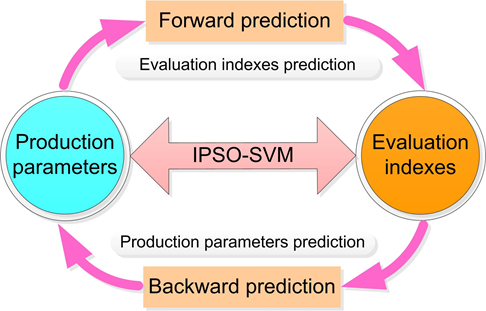Fig. 1. Bidirectional prediction model of wood fiber production

The outline of this paper is described as follows: The first section describes the research background and motivation of this paper; the second section introduces the materials and methods of experiments and the process of establishing the bi-directional prediction model of wood fiber production; the third section verifies the performance of the proposed model with the experimental data and compares the results with other homogeneous methods. Then, applications of the bi-direction model were investigated. Finally, the research conclusions are presented.

EXPERIMENTAL

Materials

Fiberboard production (Fig. 2) consists of the following sequence of steps: material preparation, chip refining, fiber drying and sizing, mat forming and prepressing, hot pressing, and fiberboard finishing (Li et al. 2007). Among these steps, the chip refining process is especially important. Figure 2 shows the main technological process of refining. The experiment was conducted at a local MDF plant with a 50-ICP refiner manufactured by Andritz Group (Graz, Austria) with two 50SC020 disks. The raw material from the hopper was fed by feeding screw to the pre-heater, where hot steam was used to heat the chips and thus soften the lignin. In this way, the energy consumption during defiberation was reduced sharply. The steaming time was determined by accumulated chip height in the pre-heater. The material from the pre-heater was transferred by conveyer screw to the center of the stationary refiner disc after the steam-softening, and hence into the refining zone. Under the combined action of the tensile force, compression force, shear force, torsion force, friction force and impact force from refining disks applied to the wood material, it was eventually separated into fibers. The fibers were unloaded through the discharge pipe at the bottom of the refiner under the steam pressure. By adjusting the opening ratio of the valve installed on the discharging pipe, it was possible to control the amount of unloaded fibers.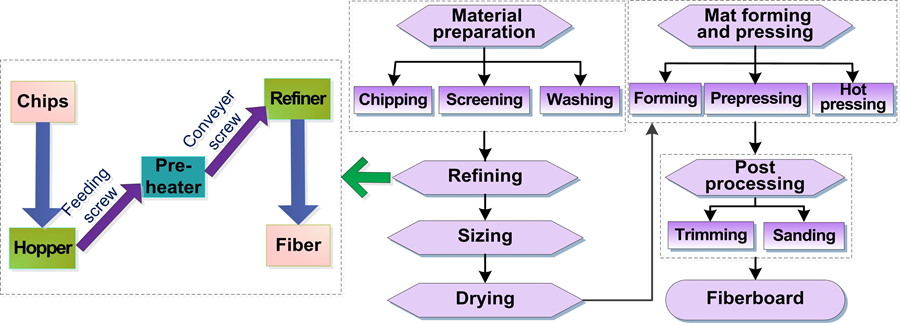Fig. 2. Principal steps in fiberboard production

In this trial, the raw material for ﬁberboard production was wood chips with the sizes of 2 to 10 mm in thickness and 20 to 100 mm in length and width. These wood chips were a mixture of Chinese poplar (P. lasiocarpa Oliv.) and Chinese larch (Larix potaninii). The moisture content of the wood chips was increased with the washing and steaming and was reduced with being squeezed by screws, and the final moisture content of the chips became constant at 50% before refining. The steam pressure of wood chips at the refiner’s entrance remained nearly constant, but the pressure changed slightly with the adjustment of the opening ratio of the discharge valve. The variation range of the steam pressure changed from 0.847 MPa to 0.877 MPa. The average pressure was 0.865 MPa, and the corresponding saturated steam temperature was 173.709 °C. The gap between the two refining disks was pre-set to 0.1 mm.

Fiber quality is normally assessed by screening values in practical production (Wei et al. 2013), as the length of the fiber is very important to the mechanical strength of the wood products (Garcia-Gonzalo et al. 2016). Fibers with moderate ratios of length/width in shape are vital for the quality of MDF. Based on mill practices, fibers of sizes from 20 to 120-screen mesh were considered as qualified fibers. Fibers smaller than 120 mesh were too small, and they consumed excessive energy.

The energy consumption was denoted with specific energy consumption (SEC, power consumption for per ton dry fibers); the fiber quality was denoted with percentage of qualified fibers (PQF) in total amount of fibers, calculated as follows:

PQF (%) = mass of qualified fiber (g) / 10 g × 100 (1)

For each measurement, 10 g fibers were collected off the production line every 2 h and screened into qualified fibers with a fiber classifier. Data including content of Chinese poplar (CP), accumulated chip height (CH), conveyer screw revolution speed (SR), opening ratio of the discharge valve (OV), and the changes in SEC were monitored and recorded every 2 h by sensors installed on the production line.

A bi-directional prediction model was established with the experimental data. For the model, the inputs were CP, CH, SR, and OV, and the outputs were PQF and SEC in the forward prediction. These sets of parameters were switched for the backward prediction.

Methods

The SVM model

Proposed by Vapnik (1999), SVM is a promising new classification and regression algorithm. Due to both powerful intelligent leaning capability and solid statistical theoretical foundation (Cao et al. 2017; Tang et al. 2017), the SVM possesses excellent prediction performance for the indeterminate and nonlinear relationship between the refining parameters and evaluation indexes. Based on the structural risk minimization principle, SVM achieves an optimum network structure. The structure of SVM is shown in Fig. 3.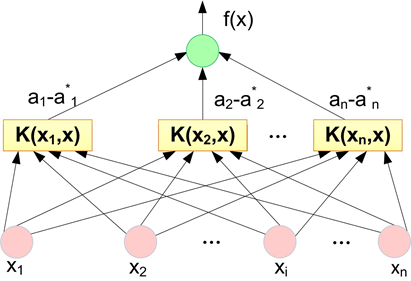Fig. 3. The structure of support vector machine

The SVM is exhibited as a three-layered network structure (Chu et al. 2017), where xi is the input data, f(xi) is the output data, and K(xi, xj) is kernel function. The radial basis function (RBF) was chosen as the kernel function due to its good properties of nonlinear forecast limited number of parameters (Keerthi and Lin 2003). The performance of SVM depends on two important parameters, i.e., the penalty factor “C” and the width of the RBF kernel “σ”. In this study, IPSO was used to optimize both parameters of the SVM model.

Overview of particle swarm optimization and its improvement methods

The PSO algorithm is an evolutionary computation algorithm that is inspired by the behavior of birds flocking (Kennedy and Eberhart 1995). PSO obtains the optimal solution of space through iterative optimization in the D-dimension search space. The particles’ speed (V) and location (X) are calculated and updated by tracking two target values (individual extremum and global optimal solution) according to Eq. 2 and Eq. 3,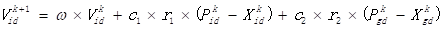(2)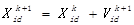(3)

where ω denotes the inertia weight that determines the impact of previous velocity, k denotes the current generation,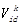and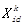denote the velocity and position of the i-th particle on dimension d (d = 1, 2, …, D), respectively,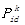and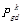denote its best individual position and global position on dimension d, c1 and c2 are personal and social learning factors, and r1 and r2 are two random numbers distributed uniformly in the range [0,1].

Although PSO has been applied to many practical problems, it still suffers from premature convergence and poor quality of solution in the case of small amount of training samples. To overcome the shortcomings, the algorithm can adaptively modify the parameters of ωc1, and c2 that have important influence on the optimization effects of PSO algorithm according to the number of iterations as follows,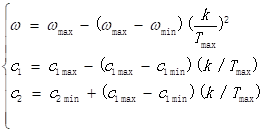(4)

where Tmax is the maximum generation, ωmin and ωmax respectively represent the minimum and maximum inertia weights, and c1minc2min, c1max, and c2max represent the minimum and maximum learning factors, i.e., ω∈[ωmin, ωmax], c1∈[c1min, c1max], and c2∈[c2min, c2max].

SVM based on the IPSO

The IPSO can automatically determine the parameters of SVM and control the predictive accuracy and generalization ability simultaneously. The overall framework of the proposed IPSO-SVM forecasting model is shown in Fig. 4 and the details of optimizing the parameters of the SVM model based on IPSO are described as follows:

Step 1: Initializing parameters

Initialized IPSO parameters including the particle population, the maximum generation, the rage of ωc1, and c2 and the space of the particles [Xi(1), Xi(2)], which represents the parameters [C, σ] of the SVM model. Besides, to alleviate the adverse effect of over-ﬁtting phenomenon, the parameter k for k-fold cross-validation technique needs to be determined in IPSO algorithm.

Step 2: Initializing the particle swarm.

Set gen = 0, randomly initialize the location X and flight velocity V of particle swarm for each individual particle i, and calculate the initial fitness with the beginning location and velocity. Copy the fitness and position vector of each particle to itself memory fitness and memory position vector, respectively.

Step 3: The iterative optimization

The velocity and location of each individual particle were updated according to Eq. 2 to 4. The individual optimal value and the global optimal value were updated based on the fitness of new particle and store the X𝑖d and X𝑔d at the current iteration. Set gen = gen + 1.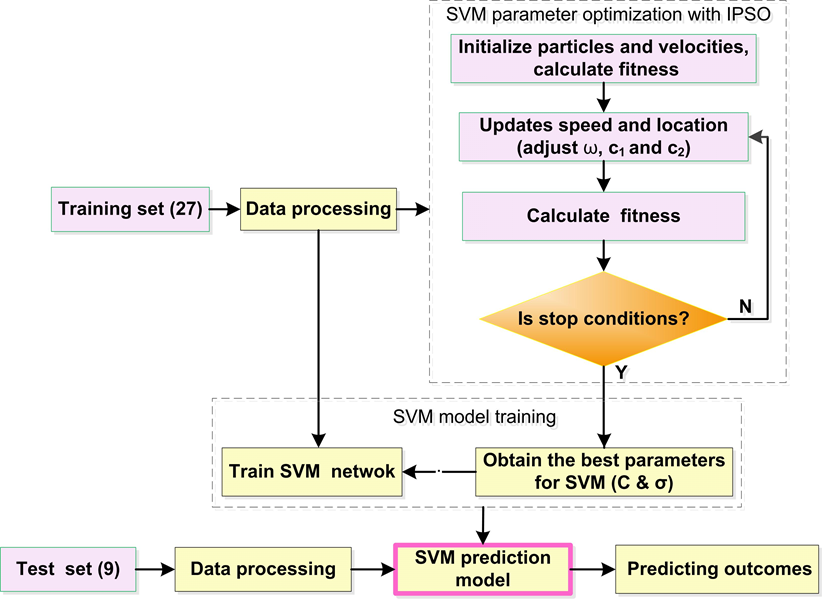Fig. 4. Diagram of the proposed IPSO-SVM forecasting model

Step 4: Circulation stops

If the stop condition, i.e., the maximum number of iterations or preset accuracy is reached, is met, then the location of the global optimal particle (the best C and the best σ) is obtained and inputted into the SVM model for training; otherwise, go back to Step 3.

The preprocessing of sample data

After eliminating singular data to reduce the possibility of overfitting, 36 groups of data were used as sample, in which 27 groups of data were selected as training set to establish the models, while the remaining 9 groups of data were chosen as test set to assess the prediction capability and robustness of the model. In order to ensure the training stability of the models and avoid the negative influence caused by discrepancy of quantitative dimension, the preprocessing of sample data should be implemented firstly. The data is normalized according to the following formulas.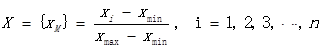(5)

In Eq. 5, xM is the normalized data; xi is the original data from the experiment; n is the number of each variable; xmax and xmin denote the maximum and the minimum raw input and output values, respectively. The original data are normalized to the range of 0 to 1.

In order to examine the performance of the new prediction models, the proposed IPSO-SVM model was compared to the back propagation neural networks (BPNN), radial basis function neural networks (RBFNN), SVM and PSO-SVM, respectively, with the test set. After several independent trials, two neuron numbers in the hidden layer of BPNN were set as 10 and 12, and the expansion speed of RBF in RBFNN was set as 35. The necessary initialization parameters of the other methods are presented in Table 1.

Table 1. Values of the Parameters Involved in the Algorithms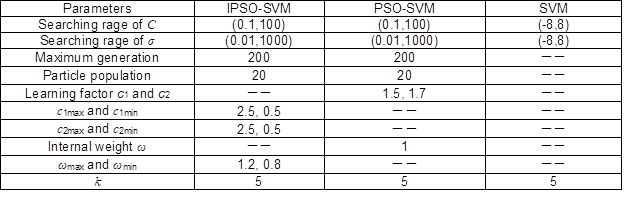Additionally, assessment indicators were the mean absolute error (MAE), root mean square error (RMSE), mean relative error (MRE), and Theil’s inequality coefficient (TIC). They are defined according to the following formulas,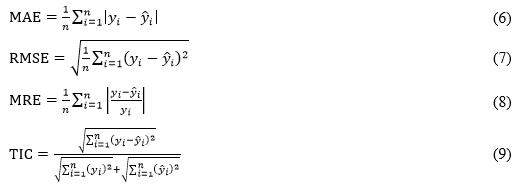where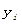are the actual outputs (experimental data);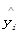are the outputs of models (predicted data); and n is the number of compounds in the analyzed data set.

RESULTS AND DISCUSSION

Forward Prediction

In forward prediction, the parameters of fiber production were set as inputs, while corresponding evaluation indexes as outputs. The best parameters for SVM were gained through optimizing them based on the IPSO algorithm, and then the forward prediction model was established based on SVM with 18 support vectors. Figure 5 shows the comparison curves between actual and predicted values of training sample set. The predicted values had a high level of agreement with the actual data. The relative error of the IPSO-SVM model based on training set for forward prediction is shown in Fig. 6. For PQF and SEC, the maximum relative errors were 5.86% and 3.36%, and the MRE were 1.37% and 1.04%, respectively. Thus, the proposed IPSO-SVM model has high accuracy and good performance.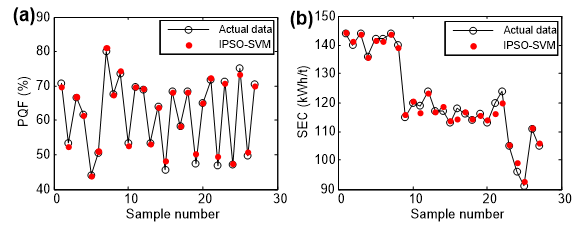Fig. 5. Training results of the IPSO-SVM model for forward prediction: (a) PQF, and (b) SEC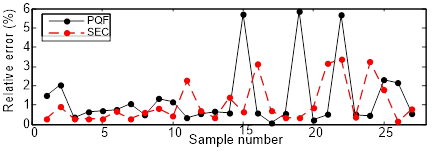Fig. 6. The relative error of the IPSO-SVM model based on training set for forward prediction

To further elaborate the superiority of the proposed model in terms of the forward prediction, based on the test set, the performances of several methods, including IPSO-SVM model, SVM, PSO-SVM, and other commonly used soft computing techniques, such as BPNN, RBFNN, were compared.

Figure 7 gives the test errors of these methods. For PQF, the maximum errors of BPNN, RBFNN, SVM, PSO-SVM, and IPSO-SVM were 10.43%, -7.80%, 6.40%, 2.68%, and -2.21%, respectively; while for SEC of those were 15.49 kWh/t, 18.20 kWh/t, 9.91 kWh/t, 6.46 kWh/t, and 5.53 kWh/t, respectively. Obviously, the proposed IPSO-SVM had the minimum absolute errors.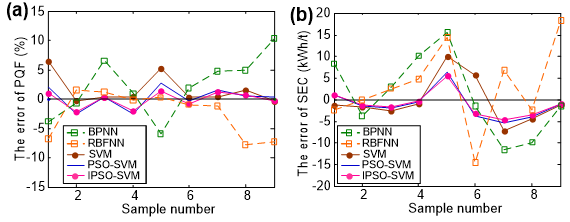Fig. 7. Test errors of the models based on BPNN, RBFNN, SVM, PSO-SVM and IPSO-SVM: (a) PQF, and (b) SEC

The detailed errors are listed in Table 2. Compared with the BPNN, RBFNN, SVM and PSO-SVM methods, MAE of IPSO-SVM decreased by 68.97%, 65.14%, 35.51%, and 16.31%, respectively. Similarly, RMSE decreased by 68.94%, 68.97%, 44.14%, and 17.71%, MRE decreased by 71.98%, 65.84%, 36.66%, and 17.95%, and TIC decreased by 70.64%, 69.25%, 46.52% and 19.61%, respectively. IPSO-SVM had better predictive performance than the other methods for the forward prediction of fiber production.

Table 2. Comparison of Errors among the Models based on BPNN, RBFNN, SVM, PSO-SVM and IPSO-SVM Methods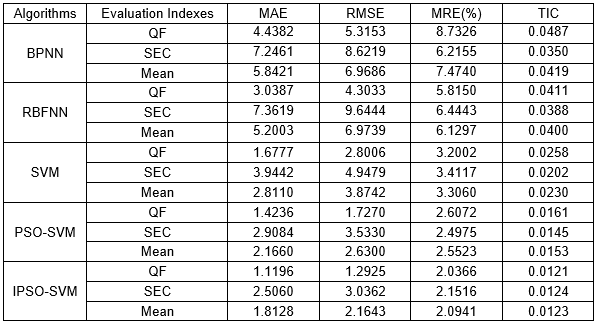Backward Prediction

The backward prediction is the reverse process of the forward prediction. Thus, evaluation indexes were set as inputs and production parameters as outputs for the backward prediction. The best parameters for SVM were obtained by optimizing them based on the IPSO algorithm, and then the backward prediction model was established based on SVM with 22 support vectors. Figure 8 shows the comparison curves between actual and predicted values of training set. The predicted values of the training set matched the experimental data well in general. Figure 9 shows the relative errors of the IPSO-SVM model based on the training set for the backward prediction. For CP, CH, SR, and OV, the maximum relative errors were 1.79%, 3.70%, 10.12%, and 7.25%, and the MRE were 0.91%, 0.61%, 1.43%, and 2.13%, respectively. The new model established by the IPSO-SVM algorithm clearly had promising prediction ability.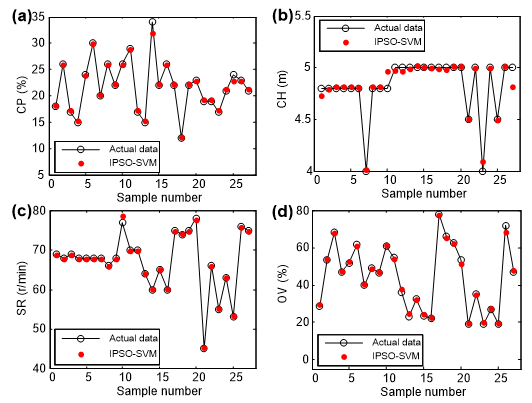Fig. 8. Training results of the IPSO-SVM model for backward prediction: (a) CP, (b) CH, (c) SR, and (d) OV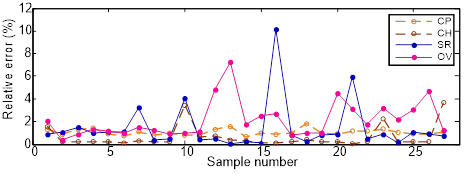Fig. 9. The relative errors of the IPSO-SVM model based on training set for backward prediction

Errors for the IPSO-SVM were also compared to those of BPNN, RBFNN, SVM and PSO-SVM based on the test set, which is shown in Fig. 10. For CP, the maximum errors of BPNN, RBFNN, SVM, PSO-SVM and IPSO-SVM were 5.43%, -4.20%, -1.58%, 0.23%, and 0.23%; for CH, they were -0.35 m, -0.43 m, -0.20 m, -0.14 m, and -0.11 m; for SR, they were 22.83 r/min, 12.91 r/min, 6.57 r/min, 4.15 r/min, and 3.87 r/min; and for OV, they were -12.88%, -17.25% -12.97%, 5.83%, and 4.03 %, respectively.